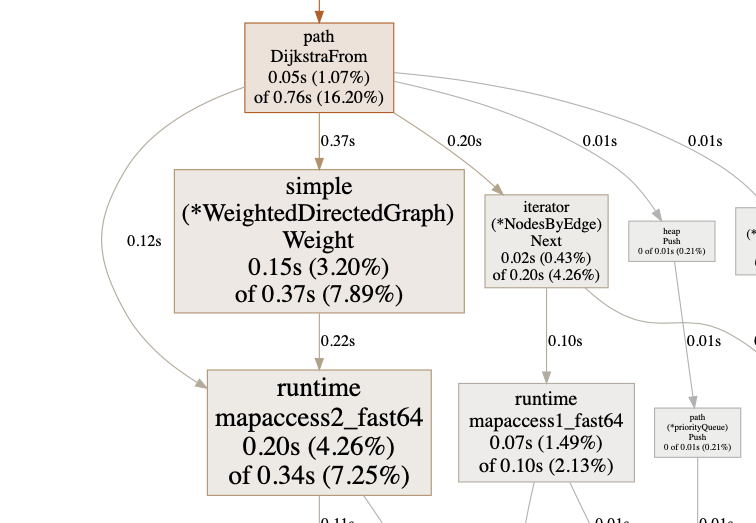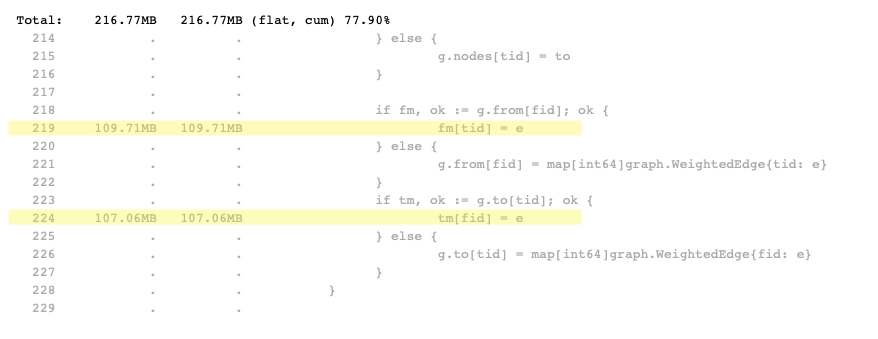This is a walk-through on how to debug and fix performance issues in golang.

## The story

Once, I had a task to implement a graph and to find the shortest path between two node. I found this two libraries for graph implementation

While gonum is a very well renowned library, the implementation from yourbasic is more like a demo or prove of concept. Let’s see how these two performed for my tasks.

To get started I created a very simple program to test which one can get me the shortest path in shortest time for 1000 nodes.

``````...
func main() {
fmt.Print("Begin graph test\n")
rand.Seed(time.Now().UnixNano())
noOfNodes := 1000
fmt.Print("Creating the ping times table...")
pingTimes := createPingTimes(noOfNodes)
fmt.Print("Done.\n")

from, to := int64(rand.Intn(noOfNodes)), int64(rand.Intn(noOfNodes))

fmt.Print("=== basic graph ===\nBuilding graph...")
sg := yourBasicGraphBuild(pingTimes)
fmt.Print("Done.\nTesting shortest path...\n")
yourBasicGraphPath(sg, from, to)
fmt.Print("Done.\n")
fmt.Print("=== gonum graph ===\nBuilding graph...")
gg := gonumGraphBuild(pingTimes)
fmt.Print("Done.\nTesting shortest path...\n")
gonumGraphPath(gg, from, to)
fmt.Print("Done.\n")
}
...
``````

build, run, and the result was:

``````Begin graph test
Creating the ping times table...Done.
=== basic graph ===
Building graph...Done.
Testing shortest path...
time: 15.433212 ms,from 193, to 226, path [193 739 226], distance: 12
Done.
=== gonum graph ===
Building graph...Done.
Testing shortest path...
time: 141.207139 ms,from 193, to 226, path [193 739 226], distance: 12
Done.
``````

Both implementations are using Dijkstra algorithm but the implementation from yourbasic is ten times faster than the gonum implementation.

In the next section we will find out why is such a big difference.

## The fun part, debugging

I started by creating a benchmark test for each of the implementations

file: graph_test.go

``````...
func BenchmarkYourBasicGraph1000(b *testing.B) {
g := createYourBasicGraph(1000)
var from, to int64
from = int64(rand.Intn(195) + 5)
for {
to := int64(rand.Intn(195) + 5)
if from != to {
break
}
}
for n := 0; n < b.N; n++ {
_, _ = basicgraph.ShortestPath(g, int(from), int(to))
}
}
...
func BenchmarkGonumGraph1000(b *testing.B) {
g := createGonumGraph(1000)
var from, to int64
from = int64(rand.Intn(195) + 5)
for {
to := int64(rand.Intn(195) + 5)
if from != to {
break
}
}
for n := 0; n < b.N; n++ {
pth := path.DijkstraFrom(simple.Node(from), g)
_, _ = pth.To(to)
}
}
...
``````

and run the tests

``````go test -cpu 8 -benchmem -run=^\$ -bench . graph_test.go
``````

and the result is

``````goos: darwin
goarch: amd64
cpu: Intel(R) Core(TM) i9-9880H CPU @ 2.30GHz
BenchmarkYourBasicGraph1000-8                 72          15834949 ns/op         1293871 B/op       1936 allocs/op
BenchmarkGonumGraph1000-8                      3         358852355 ns/op        169655304 B/op    868938 allocs/op
PASS
ok      command-line-arguments  5.532s
``````

Now I can see that gonum implementation is worst on all 3 indicators: ns/op , B/op and allocs/op

This benchmark is only reading the nodes, but what about the memory consumption to the whole graph

file: graph_test.go

``````...
func TestYourBasicGraphMemory(t *testing.T) {
var m, m2 runtime.MemStats
runtime.GC()
createYourBasicGraph(1000)
totalBytes := m2.Alloc - m.Alloc
t.Logf("Memory usage %s, %s, %s", byteCountIEC(m.Alloc), byteCountIEC(m2.Alloc), byteCountIEC(totalBytes))
}
...
func TestGonumGraphMemory(t *testing.T) {
var m, m2 runtime.MemStats
runtime.GC()
createGonumGraph(1000)
totalBytes := m2.Alloc - m.Alloc
t.Logf("Memory usage %s, %s, %s", byteCountIEC(m.Alloc), byteCountIEC(m2.Alloc), byteCountIEC(totalBytes))
}
...
``````

run the tests again

``````go test -v .
``````

and get the results

``````=== RUN   TestYourBasicGraphMemory
graph_test.go:84: Memory usage 231.5 KiB, 40.8 MiB, 40.5 MiB
--- PASS: TestYourBasicGraphMemory (0.11s)
=== RUN   TestGonumGraphMemory
graph_test.go:111: Memory usage 239.2 KiB, 246.6 MiB, 246.3 MiB
--- PASS: TestGonumGraphMemory (0.76s)
PASS
ok      graphtest    1.142s
``````

Interesting! The gonum implementation is using 246Mb of memory for 1000 nodes, while the yourbasic implementation is using only 40 Mb.

Next part is to profile the code

``````go test -run=^\$ -bench=^BenchmarkGonumGraph1000\$ -cpuprofile=cpu-gonum.p
go tool pprof -http=:8080 cpu-gonum.p
``````

and if you select only where we search for the shortest path `path.DijkstraFrom` you will see that the runtime spends most of time by reading from the mapsJust a side note: “if you don’t understand the implementation and if you have no idea what the code does, you will never have a chance to understand the profile graph”

Let’s look at the source code to see how the WeightedDirectedGraph is implemented

``````type WeightedDirectedGraph struct {
nodes map[int64]graph.Node
from  map[int64]map[int64]graph.WeightedEdge
to    map[int64]map[int64]graph.WeightedEdge

self, absent float64

nodeIDs *uid.Set
}
``````

There are 5 maps in this struct and this why is spending so much time on map lookup, after all we have 999.000 edges to search for.

Also this maps takes quite a lot of memory. At least 16 bytes for keys and another 24 bytes for `WeightedEdge` all this multiplied by the number of edges.

Let’s use the memory profiles to see what we can find

``````go test -run=^TestGonumGraphMemory\$ -memprofile=mem-gonum.p
go tool pprof -http=:8080 mem-gonum.p
``````You can see here there are huge allocations where edges are added to the from and to map, and this is the cause for the memory issue that we had.

On the other hand the implementation provided by yourbasic/graph is using only one slice of maps, the `ID()` of the node will be the index of the node, and this is what we will use next.

The only way to improve this is to provide our own implementation of the directed graph. Let’s name it LightWeightedGraph

``````type LightWeightedGraph struct {
edges []map[int64]float64
}
``````

Now, in order to be able to use this struct with gonum we need to implement some interfaces

file: graph.go

``````...
func (wg LightWeightedGraph) Nodes() graph.Nodes {
return NewNodeIterator(len(wg.edges))
}

func (wg LightWeightedGraph) Node(id int64) graph.Node {
return simple.Node(id)
}

func (wg LightWeightedGraph) From(id int64) graph.Nodes {
from := wg.edges[id]
return NewNodes(from)
}

func (wg LightWeightedGraph) Weight(xid, yid int64) (w float64, ok bool) {
if xid == yid {
return 0, true
}
if e, ok := wg.edges[xid][yid]; ok {
return e, true
}
return 0, false
}

func (g LightWeightedGraph) SetWeightedEdge(e graph.WeightedEdge) {
var (
from = e.From()
fid  = from.ID()
to   = e.To()
tid  = to.ID()
)
if fid == tid {
}

if g.edges[fid] == nil {
g.edges[fid] = make(map[int64]float64, 1)
}
g.edges[fid][tid] = e.Weight()
}
...
``````

The challenging part is that the library is using a node iterator to retrieve the nodes and the neighbors, we need to implement this too.

file: graph.go

``````...
type NodeIterator struct {
len     int
current int
}

func NewNodeIterator(len int) *NodeIterator {
return &NodeIterator{
len:     len,
current: -1,
}
}

func (i *NodeIterator) Next() bool {
if i.current >= i.len-1 {
return false
}
i.current++
return true
}

func (i *NodeIterator) Len() int {
return i.len - i.current
}

func (i *NodeIterator) Reset() {
i.current = -1
}
...
``````

This is a very simple node iterator that does nothing but to return the index of the node as id of the node.

The iterator for the `From(id int64)` function is a little more complicated because we need to provide an iterator over a map keys. Luckily in the gonum/graph source code I found a very nice implementation of a map iterator. The bad news is that we cannot use it, so I just copied.

file: map.go

``````type Nodes struct {
nodes int
iter  *mapIter
pos   int
curr  graph.Node
}

func NewNodes(nodes map[int64]float64) *Nodes {
return &Nodes{nodes: len(nodes), iter: newMapIterNodes(nodes)}
}
...
``````

Let’s update our main file and the `gonumGraphBuild` function

file: main_after.go

``````func gonumGraphBuild(pingTimes [][]int64) graph.Graph {
g := NewLightWeightedGraph(len(pingTimes))
for i := 0; i < len(pingTimes); i++ {
for j := 0; j < len(pingTimes[i]); j++ {
if j != i {
e := simple.WeightedEdge{F: simple.Node(i), T: simple.Node(j), W: float64(pingTimes[i][j])}
g.SetWeightedEdge(&e)
}
}
}
return g
}
``````

and we run the test again

``````go run .
``````

and the result is

``````Begin graph test
Creating the ping times table...Done.
=== basic graph ===
Building graph...Done.
Testing shortest path...
time: 15.727268 ms,from 725, to 172, path [725 172], distance: 6
Done.
=== gonum graph ===
Building graph...Done.
Testing shortest path...
time: 79.618552 ms,from 725, to 172, path [725 172], distance: 6
Done.
``````

Not bad. By providing a custom implementation we managed reduce the running time by half. But still this is not as fast as the yourbasic implementation even if we did used exactly the same storage for the graph. It look like this is the overhead of using the `iterator` and a bigger data types `int64` and `float64`

I had a dispute once about whether is the best to use a custom implementation vs to use a library.

This is the good example that is not always the best to use a library, and this is why:

1. the library has a different goal than yours. For gonum library, the graph part, it easy to see that the goal is to cover as much as possible of the all known algorithms. A side effect of this is that the interfaces contains more functions that you need and the implementations contains more fields than is required.

For example the `type WeightedDirected interface` contains `To(id int64) Nodes` function which is not used by the Dijkstra implementations but is used by the `Louvain` implementation for community detection and the field `to map[int64]map[int64]graph.WeightedEdge`was added to the type`WeightedDirectedGraph` just to fulfill this requirement.

``````type WeightedDirectedGraph struct {
nodes map[int64]graph.Node
from  map[int64]map[int64]graph.WeightedEdge
to    map[int64]map[int64]graph.WeightedEdge // I don't need this

self, absent float64

nodeIDs *uid.Set // I don't need this
}
``````

2. you will spend a huge amount of time to understand how the library was structured, and why was structured, and in the end you might end up at the conclusion that the library will only solve half of the problem.

3. you will be forced to implement functions that you don’t need and you don’t use, and for some of them you will have to use

``````panic("not implemented")
``````

which makes you code very ugly

In the end I chose to copy the implementation from yourbasic into my project and to adjust it to my needs.

## Conclusion

The pprof tool for go is very powerful and it can help you troubleshoot performance and memory issues.

This are some articles that I used as inspiration for this post.

1712 Words

2021-04-22 20:22 +0000